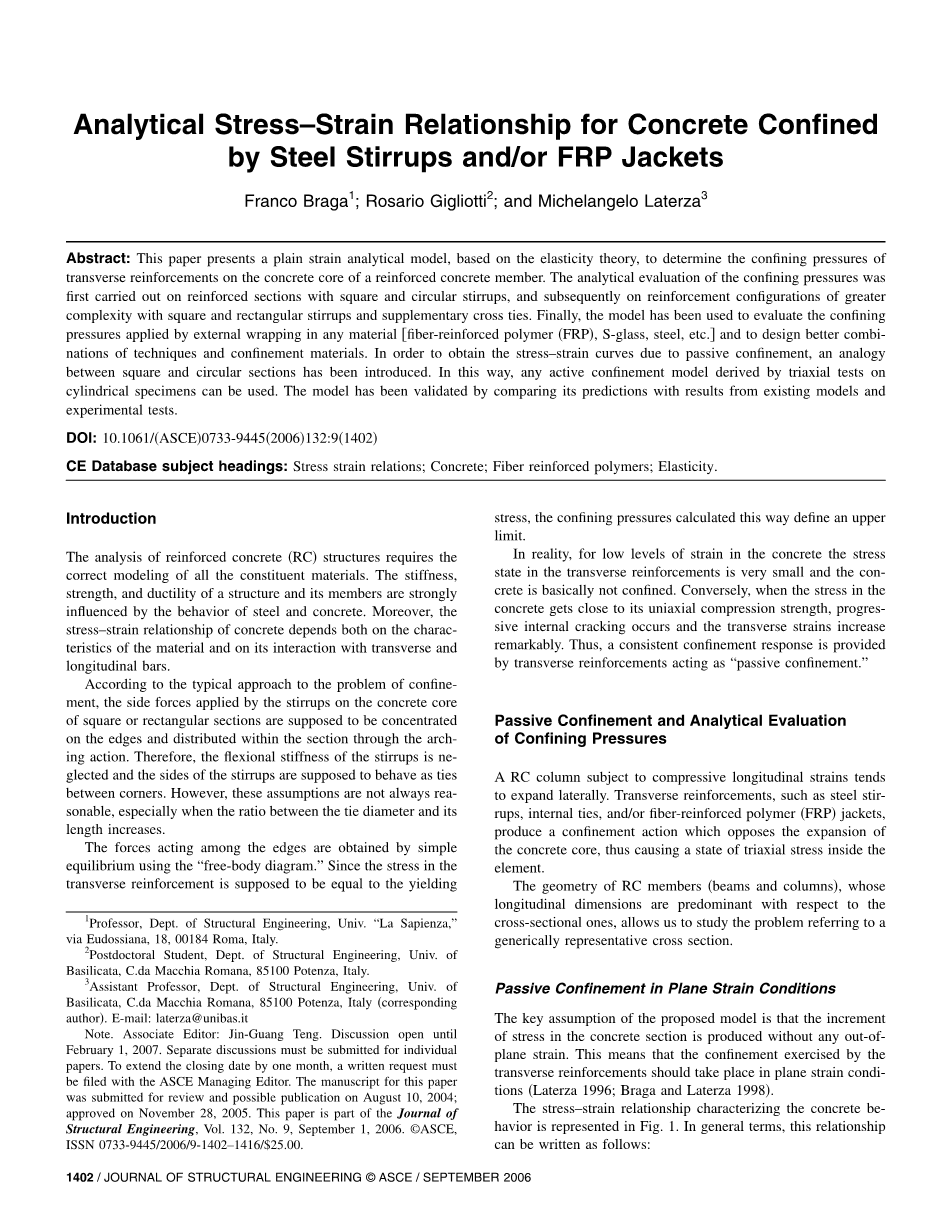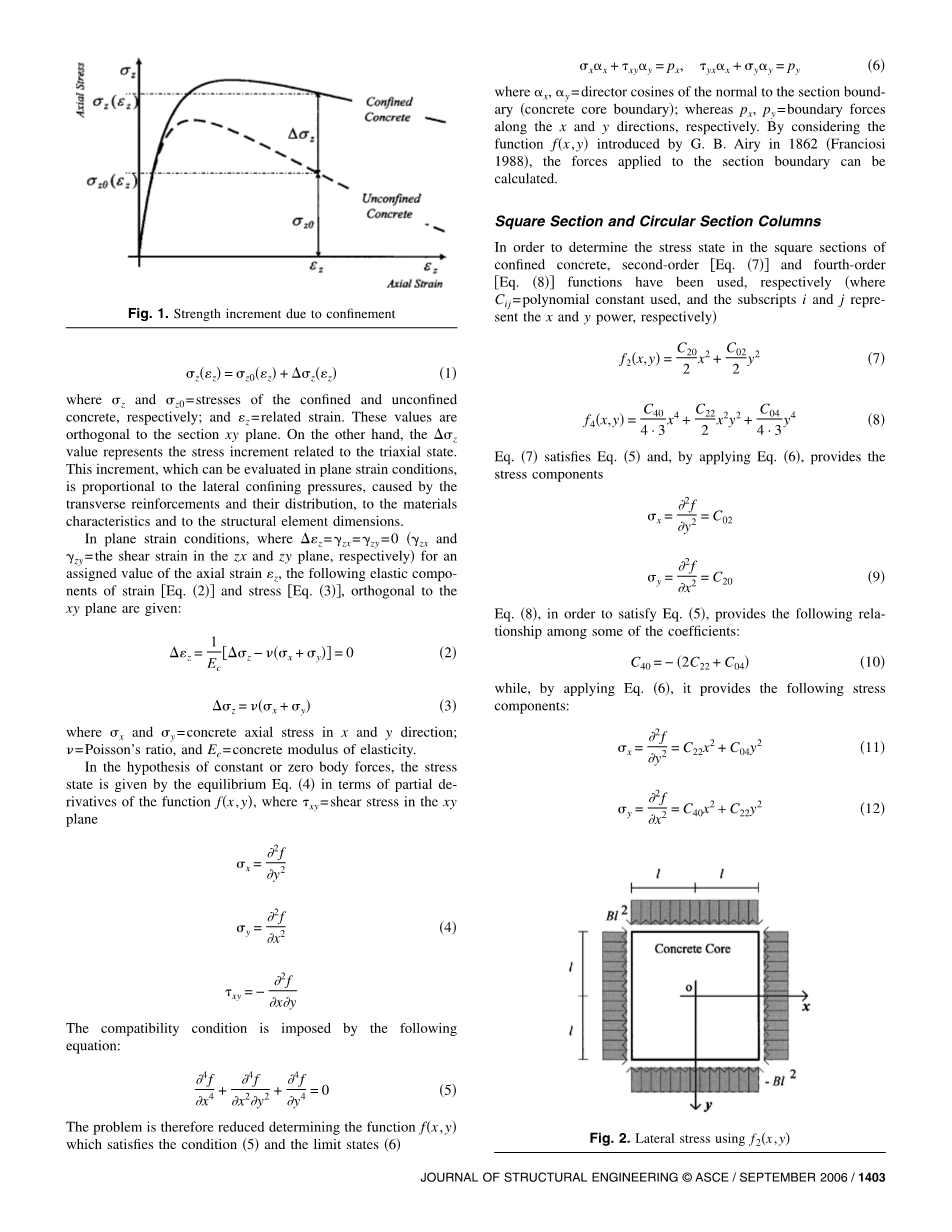# 钢箍筋和/或FRP夹套约束混凝土的应力-应变分析关系外文翻译资料

2022-08-05 02:08CE数据库主题：应力应变关系；混凝土；纤维增强聚合物；弹性。

# 被动约束与围压分析评价

## 平面应变条件下的被动约束

zz=z0z zz 1

1

 z=zminus;x y=0 Ec 2 z=x y 3

4 x22 y4=0.5y

xx xyy= px, yxx yy= py6 其中x，y=垂直于截面边界混凝土核心边界的方向余弦；而px，py=分别沿x和y方向的边界力。通过考虑函数fx，y 由G.B.Airy于1862年引入，franciosi1988，可以计算作用在截面边界上的力。

### 方形截面柱和圆形截面柱

C

f2x,y = 20x2 C02y2 7

f4x,y = C40 x4 C22x2y2 C04 y4 8

4 · 3 2 4 · 3

2f

x=y2 = C02

2f

y=x2 = C20

9

C40 = minus; 2C22 C04 10

 2f x=2 = C222 C042xy y 11 2f y=2 = C402 C222xy 12

 图3。横向应力f4x，y
 xy= minus;= minus; 2C22xy xy 通过假设，因为几何对称 13 C20 =C02=minus;Bl2 此后，导出所报告的表达式15 2f2 x=2 = minus; Bl y 14 2f y=2 = minus; Bl2 15

2

2f

xy== 0

## xy

C40=C04=minus;A，C22=A，可获得表示沿边界线抛物线变化的牵引力和线性变化的切向应力的应力表达式16，如图3所示

### x= Axminus; y minus; Bl, = Ayminus; x minus; Bl, = minus; 2A xy2 22y2 22xy

17

 图4。侧向围压f2x，y和f4x，y之和

z=x y=minus;Ax2minus;y2minus;Bl2 Ay2minus;x2minus;Bl2=2Bl2

18

q

fr= n = m = 19

S

z=n m=2n=2

S

20

 1 1 x=xminus; y, y =yminus; x Ec Ec 21 2 1 xy=yx=xy 22

### Ec

21

 1 1 x=xminus; y, y =yminus; x Ec Ec 21 2 1 xy=yx=xy 22

22

### Ec

Vx=xx 21xyy, Vy=yy 21yxx23